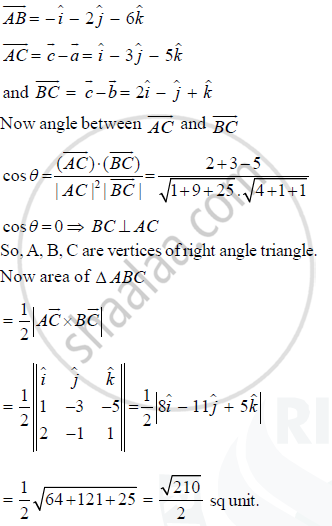Share

# Show that the Points A, B, C with Position Vectors 2hati- Hatj + Hatk, Hati - 3hatj - 5hatk and 3hati - 4hatj - 4hatk Respectively, Are the Vertices of a Right-angled Triangle. Hence Find the Area of the Triangle - CBSE (Science) Class 12 - Mathematics

ConceptIntroduction of Product of Two Vectors

#### Question

Show that the points A, B, C with position vectors 2hati- hatj + hatk, hati - 3hatj - 5hatk and 3hati - 4hatj - 4hatk respectively, are the vertices of a right-angled triangle. Hence find the area of the triangle

#### Solution

Given

veca =  2hati - hatj + hatk

vecb = hati -3hatj - 5hatk

vecc = 3hati - 4hatj - 4hatk

then

bar(AB) = vecb - vecaIs there an error in this question or solution?

#### Video TutorialsVIEW ALL 

Solution Show that the Points A, B, C with Position Vectors 2hati- Hatj + Hatk, Hati - 3hatj - 5hatk and 3hati - 4hatj - 4hatk Respectively, Are the Vertices of a Right-angled Triangle. Hence Find the Area of the Triangle Concept: Introduction of Product of Two Vectors.
S